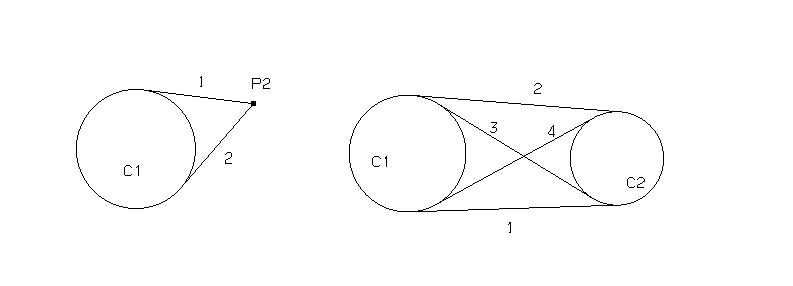```

Lines use the identification letter L ("Line").

LN PT  PT
Line between 2 points

Startpt           Startpoint of the line
Endpt             Endpoinoint of the line
[unlimited]       limited / unlimited

Example:
L1 = P1 P(100 100)

LN parallel
Line parallel to a line / vector, through a point.
For the point can be defined a X-Offset, Y-Offset and Z-Offset.

Direction         direction of the line as vector
[BasePoint]       a point on the line
[Length]          length, optional.
[X-offset]        X-offset from BasePoint
[Y-offset]
[Z-offset]
[unlimited]       limited / unlimited

Example:
# parallel to X-axis, with Y-offset:
L2=DX Y(10)

LN PT Direct. [Length,Rot.Angle,TiltAngle]

Line through point, parallel to vector / line;
rotated in the constructionplane or tilted.

Point             a point on the line
Direction         direction of the line as vector
[Length]          length, optional.
[Angle-Rot.]      Rotation angle in the constructionplane
[Angle-Tilt]      Tilt angle normal to the constructionplane
[unlimited]       limited / unlimited

Examples:
# Line from point with a fixed angle
L3=P1 ANG(45)

# Parallel to line L1 through P1
L4= P1 D(L1)

# Line from point with angle relative to the other line.
L4=P1 D(L1) ANG(90)

LN LN Dist.          (parall)
Line parallel to line spacing.

parall to Line   baseline (direction)
Dist.            Normal distance of line from baseline
[Position]       no value: left; Versionnumber
1, 2 lateral left / right; 3, 4 top / bottom

Examples:
# parallel to L10, distance 10, above:
L8=L10 VAL(10) MOD(3)

LN LN LN [Rotate Tilt] (mid)
Line as a midline of two lines (a bisection line through the intersectionpoint).
Can be rotated and tilted.

Line 1
Line 2
[Angle-Rot.]      Rotation angle in the constructionplane
[Angle-Tilt]      Tilt angle normal to the constructionplane

Examples:
L26=L23 L24                  # angle bisector, from intersectionpoint
L27=L23 L24 ANG(90)          # angle bisector, additionally rotated

LN tangent to 2 objs",            "TNG"
Create a tangential line between 2 curve-objects.
Inputelements can be: points, circles, curves.

- get the tangential line from:
- select curve and point on that curve for a tangent or
- select vector or line (define the direction) and a curve or
- select two curves (eg 2 circles)

object 1
object 2
[solutionNr]      solution-nr
[REVers]          fwd / bwd (key Pageup / Page Down, see REV)
[unlimited]       limited / unlimited

If one object is a line or a vector:
create tangent with constant direction (parallel to line/vector).

Tangent to curve through point on this curve; one solution only; select point first
Tangent from point outside curve to curve;    n solutions;       select curve first

solutionNr / object -:Examples:
# Line tangent to a circle, parallel to the line.
L1=C1 D(L1) MOD(1)

# Line tangent to 2 circles
L5=C1 C2 MOD(1)

```